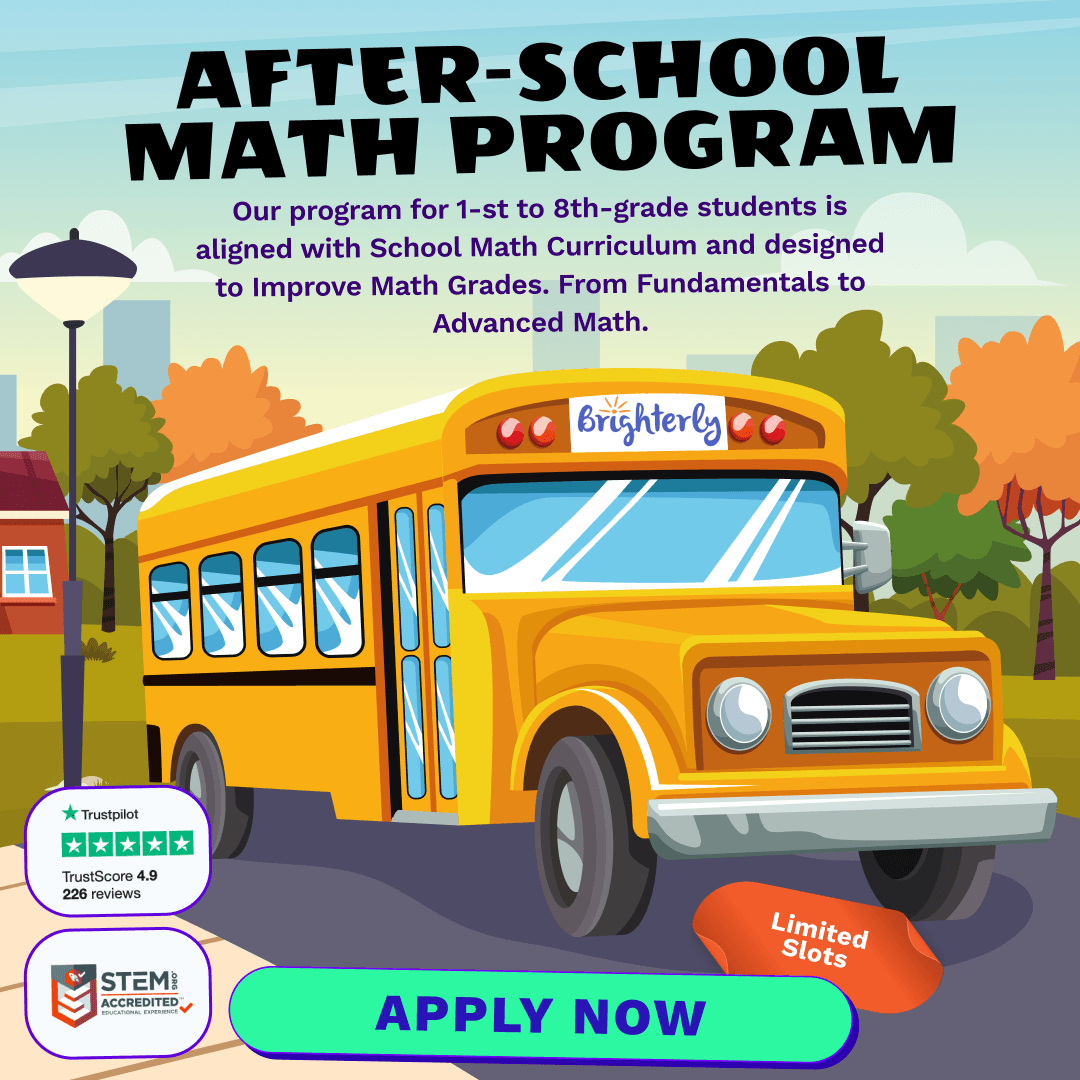# Numbers Math Practice Test for 3rd Grade – [Easy]

Welcome to Brighterly’s guide to basic numbers for 3rd grade! Numbers are the foundation of mathematics, and by the time your child reaches the 3rd grade, they’re ready to dive deeper into the fascinating world of numbers.

## Understanding Place Value

Understanding the place value of numbers is pivotal for any 3rd grader. Imagine if we didn’t know the difference between 10 and 100 – everything would be confusing! Place value tells us the value of each digit in a number, based on its position.

For instance, in the number 432:

• The digit 4 is in the hundreds place, which means its value is 400.
• The digit 3 is in the tens place, making its value 30.
• The digit 2 is in the ones place, so its value is simply 2.

By combining these values, we arrive at the original number: 432.

## Introduction to Basic Operations

At this stage, children should be comfortable with the four basic math operations:

1. Addition (+): Combining two or more numbers to get a sum.
2. Subtraction (-): Taking one number away from another.
4. Division (÷): Splitting a number into equal parts.

It’s essential to reinforce these concepts through practical applications. For instance, using real-world situations like sharing candy or adding up the scores in a game makes learning more engaging and relatable.

Check out math activities on Brighterly for more fun ways to grasp these operations.

## Exploring the World of Fractions

Fractions are a vital component of 3rd-grade math. A fraction represents a part of a whole. Imagine a pizza. If you divide it into 8 slices and take 3, you’ve taken 3/8 of the whole pizza.

In fractions:

• The numerator (the top number) tells us how many parts we have.
• The denominator (the bottom number) tells us how many parts make up a whole.

By 3rd grade, children should be familiar with simple fractions like ½, ¼, and ¾. Using visual aids like fraction games on Brighterly can make this topic more approachable.

## Conclusion

Mastering basic numbers in the 3rd grade paves the way for more advanced math topics in the future. By using engaging tools, resources, and games available on Brighterly, your child can develop a strong foundation that will benefit them throughout their academic journey. So dive in, explore, and unlock the magic of math with Brighterly!

Numbers Practice Test for 3rd Grade

Get ready for math lessons with Brighterly! Designed to reinforce foundational number concepts, this easy-level test is tailor-made for young learners ready to showcase their skills.

1 / 15

What is the value of the digit 5 in the number 583?

2 / 15

If you add 100 to the number 356, what will you get?

3 / 15

Which of the following numbers is the largest?

4 / 15

How many tens are there in the number 640?

5 / 15

If you have two quarters of a dollar, how much money do you have?

6 / 15

Which fraction represents half?

7 / 15

What comes next in the pattern: 2, 4, 6, 8, ... ?

8 / 15

If you subtract 50 from 200, what number do you get?

9 / 15

Which of the following is an even number?

10 / 15

What is the sum of 5 + 5 + 5?

11 / 15

In the number 572, which digit is in the tens place?

12 / 15

How many sides does a triangle have?

13 / 15

What number is represented by three tens and four ones?

14 / 15

Which of these is NOT a prime number?

15 / 15

If you multiply 3 by 3, what number do you get?

0%
Poor Level
Weak math proficiency can lead to academic struggles, limited college, and career options, and diminished self-confidence.
Mediocre Level
Weak math proficiency can lead to academic struggles, limited college, and career options, and diminished self-confidence.
Needs Improvement
Start practicing math regularly to avoid your child`s math scores dropping to C or even D.
High Potential
It's important to continue building math proficiency to make sure your child outperforms peers at school.

After-School Math Programs• Our program for 1st to 8th grade students is aligned with School Math Curriculum.

After-School Math Programs
Our program for 1st to 8th grade students is aligned with School Math Curriculum.